# leetcode: mini spanning tree, single src shortest path, topological

## Minimum spanning tree

A minimum spanning tree is a spanning tree with the minimum possible total edge weight in a “weighted undirected graph”.Min Cost to Connect All Points - LeetCode

You are given an array points representing integer coordinates of some points on a 2D-plane, where points[i] = [xi, yi]. The cost of connecting two points [xi, yi] and [xj, yj] is the manhattan distance between them: |xi - xj| + |yi - yj|, where |val| denotes the absolute value of val. Return the minimum cost to make all points connected. All points are connected if there is exactly one simple path between any two points.

Example 1:Input: points = [[0,0],[2,2],[3,10],[5,2],[7,0]] Output: 20 Explanation: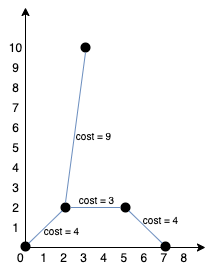We can connect the points as shown above to get the minimum cost of 20. Notice that there is a unique path between every pair of points.

Example 2: Input: points = [[3,12],[-2,5],[-4,1]] Output: 18

Constraints:

1 <= points.length <= 1000 -106 <= xi, yi <= 106 All pairs (xi, yi) are distinct.

### Kruskal

We try adding each edge, one at a time, from the lowest weight edge up to the highest weight edge. If either of the edges' vertices is not already part of the MST, then the edge is added to the MST.• code #Kruskal #unionfind
``````class Solution:
def minCostConnectPoints(self, points: List[List[int]]) -> int:
n = len(points)
root = [i for i in range(n)]
rank =  * n

def find(x):
if x != root[x]:
root[x] = find(root[x])
return root[x]

def union(x, y):
rtx = find(x)
rty = find(y)
if rtx == rty: return False
rkx = rank[rtx]
rky = rank[rty]
if rkx > rky:
root[rty] = rtx
elif rkx < rky:
root[rtx] = rty
else:
root[rty] = rtx
rank[rtx] += 1
return True

def dis(px, py):
return abs(px - py) + abs(px - py)

dis_pq = []
for i in range(n-1):
for j in range(i+1, n):
dis_pq.append((dis(points[i], points[j]), i, j)) # put all edges

heapq.heapify(dis_pq)
res = 0
while n - 1 > 0:
cur_dis, i, j = heapq.heappop(dis_pq)
if union(i, j): # if can connect, means not already connected, not a cycle
res += cur_dis
n -= 1

return res
``````

### Prim

Starting from an arbitrary vertex, Prim’s algorithm grows the minimum spanning tree by adding one vertex at a time to the tree. The choice of a vertex is based on the greedy strategy, i.e., the addition of the new vertex incurs the minimum cost.• code #Prim shorter, optimized
``````class Solution:
def minCostConnectPoints(self, points: List[List[int]]) -> int:
def dis(pi, pj):
return abs(pi - pj) + abs(pi - pj)

cost = 0
n = len(points)
visited = set()
pq = [[0, 0]] # (distance, current point)

while len(visited) < n:
shortest, cur = heapq.heappop(pq)
if cur in visited: continue

cost += shortest

for point in range(n):
if point not in visited: # from current, to every unvisited point
heapq.heappush(pq, (dis(points[cur], points[point]), point))

return cost
``````

## Single source shortest path

We often need to find the “shortest path” in a “weighted graph”.

### Dijkstra

We take the starting point u as the center and gradually expand outward while updating the “shortest path” to reach other vertices.

“Dijkstra’s Algorithm” uses a “greedy approach”. Each step selects the “minimum weight” from the currently reached vertices to find the “shortest path” to other vertices.

#### Network Delay Time - LeetCode

You are given a network of n nodes, labeled from 1 to n. You are also given times, a list of travel times as directed edges times[i] = (ui, vi, wi), where ui is the source node, vi is the target node, and wi is the time it takes for a signal to travel from source to target. We will send a signal from a given node k. Return the time it takes for all the n nodes to receive the signal. If it is impossible for all the n nodes to receive the signal, return -1.

Example 1: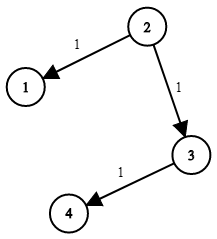Input: times = [[2,1,1],[2,3,1],[3,4,1]], n = 4, k = 2 Output: 2

Example 2: Input: times = [[1,2,1]], n = 2, k = 1 Output: 1 Example 3: Input: times = [[1,2,1]], n = 2, k = 2 Output: -1

Constraints:

1 <= k <= n <= 100 1 <= times.length <= 6000 times[i].length == 3 1 <= ui, vi <= n ui != vi 0 <= wi <= 100 All the pairs (ui, vi) are unique. (i.e., no multiple edges.)

• code wrong
``````class Solution:
def networkDelayTime(self, times: List[List[int]], n: int, k: int) -> int:
distance = [inf] * (n + 1)
distance = 0
distance[k] = 0
graph = defaultdict(list) # nei, distance
for i, j, w in times:
graph[i].append((j, w))
q = deque([k])
visited = set()
while q:
cur = q.popleft()
for nei, w in graph[cur]:
distance[nei] = min(distance[nei], distance[cur] + w)
if nei not in visited:
q.append(nei)
print(distance)
ans = max(distance)
return ans if ans != inf else -1
``````After starting point A, for neighbour B,C,D, can only choose B or C in the next round as they are the shortest, can’t choose D.

• code #dijkstra
``````class Solution:
def networkDelayTime(self, times: List[List[int]], n: int, k: int) -> int:
distance = [inf] * (n + 1)
distance = 0 # reduntant

graph = defaultdict(list) # nei, distance
for i, j, w in times:
graph[i].append((j, w))

q = [[0, k]] # distance, point

while q:
cur_dis, cur = heapq.heappop(q)
if distance[cur] != inf: continue
distance[cur] = cur_dis
for nei, w in graph[cur]:
if distance[nei] == inf:
heapq.heappush(q, (cur_dis + w, nei))
ans = max(distance)
return ans if ans != inf else -1

``````
• code
``````class Solution {
public int networkDelayTime(int[][] times, int n, int k) {
Map<Integer, List<int[]>> graph = new HashMap<>();
for (int[] time: times){
// List<int[]> nei_wei = graph.getOrDefault(time, new ArrayList<int[]>());
// nei_wei.add(new int[] {time, time});
// graph.put(time, nei_wei);
if (!graph.containsKey(time))
graph.put(time, new ArrayList<int[]>());
}
Map<Integer, Integer> dist = new HashMap<>();
PriorityQueue<int[]> pq = new PriorityQueue<>((a,b) -> a - b);
pq.offer(new int[] {0, k});
while (!pq.isEmpty()){
int[] cur_dis_node = pq.poll();
int curDis = cur_dis_node;
int cur = cur_dis_node;
if (dist.containsKey(cur)) continue;
dist.put(cur, curDis);
if (graph.containsKey(cur)){
for (int[] nei_w: graph.get(cur)){
int nei = nei_w, w = nei_w;
if(!dist.containsKey(nei)){
pq.offer(new int[]{curDis + w, nei});
}
}
}
}
if(dist.size() != n) return -1;
return dist.values().stream().max(Comparator.naturalOrder()).get();
// int res = 0;
// for (int v: dist.values()){
//     res = Math.max(v, res);
// }
// return res;
}
}

``````

#### Path With Minimum Effort - LeetCode

You are a hiker preparing for an upcoming hike. You are given heights, a 2D array of size rows x columns, where heights[row][col] represents the height of cell (row, col). You are situated in the top-left cell, (0, 0), and you hope to travel to the bottom-right cell, (rows-1, columns-1) (i.e., 0-indexed). You can move up, down, left, or right, and you wish to find a route that requires the minimum effort. A route’s effort is the maximum absolute difference in heights between two consecutive cells of the route. Return the minimum effort required to travel from the top-left cell to the bottom-right cell.

Example 1: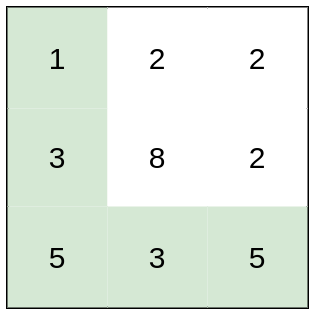Input: heights = [[1,2,2],[3,8,2],[5,3,5]] Output: 2 Explanation: The route of [1,3,5,3,5] has a maximum absolute difference of 2 in consecutive cells. This is better than the route of [1,2,2,2,5], where the maximum absolute difference is 3. Example 2: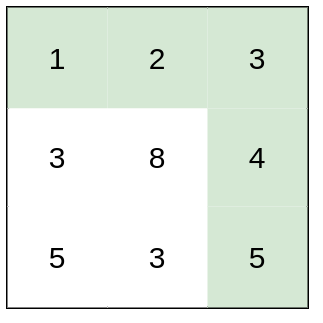Input: heights = [[1,2,3],[3,8,4],[5,3,5]] Output: 1 Explanation: The route of [1,2,3,4,5] has a maximum absolute difference of 1 in consecutive cells, which is better than route [1,3,5,3,5]. Example 3: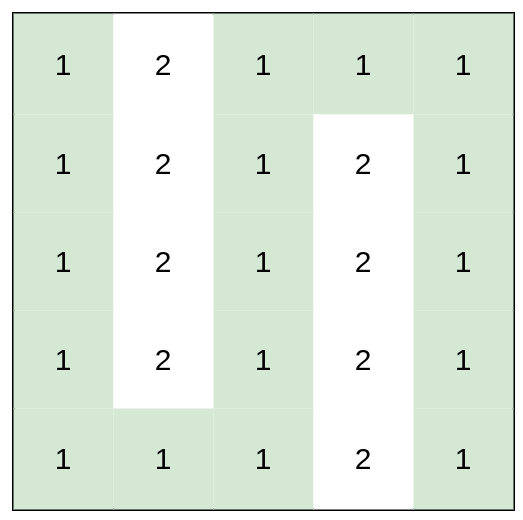Input: heights = [[1,2,1,1,1],[1,2,1,2,1],[1,2,1,2,1],[1,2,1,2,1],[1,1,1,2,1]] Output: 0 Explanation: This route does not require any effort.

Constraints:

``````rows == heights.length
columns == heights[i].length
1 <= rows, columns <= 100
1 <= heights[i][j] <= 106
``````
• code #Dijkstra as long as a new edge won’t decrease the path, i.e. diff won’t decrease, we can potentially use Dijkstra(no negative edges)
``````class Solution:
def minimumEffortPath(self, heights: List[List[int]]) -> int:
m, n = len(heights), len(heights)

diff = [[inf] * n for _ in range(m)]
diff = 0

q = [[0, 0, 0]] # max diff, (x, y)
direction = [[-1,0],[1,0],[0,1],[0,-1]]
visited = [[False] * n for _ in range(m)]
while q:
cur_diff, x, y = heapq.heappop(q)
visited[x][y] = True
for dx, dy in direction:
nx, ny = x + dx, y + dy
if 0 <= nx < m and 0 <= ny < n and not visited[nx][ny]:
newdiff = min(diff[nx][ny], max(abs(heights[nx][ny] - heights[x][y]), cur_diff))
if newdiff < diff[nx][ny]:
diff[nx][ny] = newdiff
heapq.heappush(q, [diff[nx][ny], nx, ny])

return diff[-1][-1]
``````

#### Cheapest Flights Within K Stops - LeetCode

There are n cities connected by some number of flights. You are given an array flights where flights[i] = [fromi, toi, pricei] indicates that there is a flight from city fromi to city toi with cost pricei. You are also given three integers src, dst, and k, return __the cheapest price from src to dst with at most k stops. If there is no such route, return __-1.

Example 1: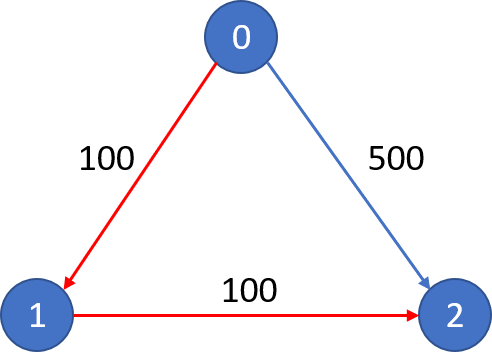Input: n = 3, flights = [[0,1,100],[1,2,100],[0,2,500]], src = 0, dst = 2, k = 1 Output: 200 Explanation: The graph is shown. The cheapest price from city 0 to city 2 with at most 1 stop costs 200, as marked red in the picture. Example 2:Input: n = 3, flights = [[0,1,100],[1,2,100],[0,2,500]], src = 0, dst = 2, k = 0 Output: 500 Explanation: The graph is shown. The cheapest price from city 0 to city 2 with at most 0 stop costs 500, as marked blue in the picture.

Constraints:

``````1 <= n <= 100
0 <= flights.length <= (n * (n - 1) / 2)
flights[i].length == 3
0 <= fromi, toi < n
fromi != toi
1 <= pricei <= 104
There will not be any multiple flights between two cities.
0 <= src, dst, k < n
src != dst
``````
• code
``````import heapq

class Solution:

def findCheapestPrice(self, n: int, flights: List[List[int]], src: int, dst: int, K: int) -> int:

# Build the adjacency matrix
adj_matrix = [[0 for _ in range(n)] for _ in range(n)]
for s, d, w in flights:

# Shortest distances array
distances = [float("inf") for _ in range(n)]
current_stops = [float("inf") for _ in range(n)]
distances[src], current_stops[src] = 0, 0

# Data is (cost, stops, node)
minHeap = [(0, 0, src)]

while minHeap:

cost, stops, node = heapq.heappop(minHeap)

# If destination is reached, return the cost to get here
if node == dst:
return cost

# If there are no more steps left, continue
if stops == K + 1:
continue

# Examine and relax all neighboring edges if possible
for nei in range(n):
if adj_matrix[node][nei] > 0:
dU, dV, wUV = cost, distances[nei], adj_matrix[node][nei]

# Better cost?
if dU + wUV < dV:
distances[nei] = dU + wUV
heapq.heappush(minHeap, (dU + wUV, stops + 1, nei))
elif stops < current_stops[nei]:
#  Better steps?
heapq.heappush(minHeap, (dU + wUV, stops + 1, nei))

current_stops[nei] = stops

return -1 if distances[dst] == float("inf") else distances[dst]
``````

### Bellman-Ford (SPFA)

“Bellman-Ford algorithm” is only applicable to “graphs” with no “negative weight cycles”.

Instead of choosing among any untraversed edges, as one does by using the “Bellman-Ford” algorithm, the “SPFA” Algorithm uses a “queue” to maintain the next starting vertex of the edge to be traversed. Only when the shortest distance of a vertex is relaxed(updated) and that the vertex is not in the “queue”, we add the vertex to the queue. We iterate the process until the queue is empty. At this point, we have calculated the minimum distance from the given vertex to any vertices.

Notice a node can be added to the queue many times, just like in Bellman-Ford algorithm we need to run many rounds until the pre matrix equals to the current matrix.

Cheapest Flights Within K Stops - LeetCode

There are n cities connected by some number of flights. You are given an array flights where flights[i] = [fromi, toi, pricei] indicates that there is a flight from city fromi to city toi with cost pricei. You are also given three integers src, dst, and k, return __the cheapest price from src to dst with at most k stops. If there is no such route, return __-1.

Example 1:Input: n = 3, flights = [[0,1,100],[1,2,100],[0,2,500]], src = 0, dst = 2, k = 1 Output: 200 Explanation: The graph is shown. The cheapest price from city 0 to city 2 with at most 1 stop costs 200, as marked red in the picture. Example 2:

Constraints:

``````1 <= n <= 100
0 <= flights.length <= (n * (n - 1) / 2)
flights[i].length == 3
0 <= fromi, toi < n
fromi != toi
1 <= pricei <= 104
There will not be any multiple flights between two cities.
0 <= src, dst, k < n
src != dst
``````

• code #bellmanford
``````class Solution:
def findCheapestPrice(self, n: int, flights: List[List[int]], src: int, dst: int, k: int) -> int:
pre = [inf] * n
pre[src] = 0
cur = pre[:]
for _ in range(k + 1):
for begin, end, cost in flights:
cur[end] = min(cur[end],  pre[begin] + cost)
if pre == cur: break
pre = cur[:]
return cur[dst] if cur[dst] != inf else -1
``````

## Topological sorting (Kahn)

Topological sorting - Wikipedia

How can we arrange the order of the courses adequately while considering prerequisite relationships between them?“Topological sorting” provides a linear sorting based on the required ordering between vertices in directed acyclic graphs.

“Topological sorting” only works with graphs that are directed and acyclic. There must be at least one vertex in the “graph” with an “in-degree” of 0.

#### Course Schedule II - LeetCode

There are a total of numCourses courses you have to take, labeled from 0 to numCourses - 1. You are given an array prerequisites where prerequisites[i] = [ai, bi] indicates that you must take course bi first if you want to take course ai.

For example, the pair [0, 1], indicates that to take course 0 you have to first take course 1.Return the ordering of courses you should take to finish all courses. If there are many valid answers, return any of them. If it is impossible to finish all courses, return an empty array.

Example 1: Input: numCourses = 2, prerequisites = [[1,0]] Output: [0,1] Explanation: There are a total of 2 courses to take. To take course 1 you should have finished course 0. So the correct course order is [0,1]. Example 2: Input: numCourses = 4, prerequisites = [[1,0],[2,0],[3,1],[3,2]] Output: [0,2,1,3] Explanation: There are a total of 4 courses to take. To take course 3 you should have finished both courses 1 and 2. Both courses 1 and 2 should be taken after you finished course 0. So one correct course order is [0,1,2,3]. Another correct ordering is [0,2,1,3]. Example 3: Input: numCourses = 1, prerequisites = [] Output: 

• code
``````class Solution {
public int[] findOrder(int numCourses, int[][] prerequisites) {
HashMap<Integer, List<Integer>> adja = new HashMap();
int[] indegree = new int[numCourses];
for(int[] edge: prerequisites){
int start = edge;
int end = edge;
indegree[end] ++;
List<Integer> nei = adja.getOrDefault(start, new ArrayList<Integer>());

}

for (int i=0; i < numCourses; ++i){
if (indegree[i] == 0){
q.offer(i); // add to last
}
}

int[] res = new int[numCourses];
int added = 0;
while(!q.isEmpty()){
int cur = q.poll(); // pop first
if (added == numCourses){
return res;
}
for (int nei: adja.get(cur)){
if (--indegree[nei] == 0){
q.offer(nei);
}
}
}
}
return new int;
}
}
``````
• code
``````class Solution:
def findOrder(self, numCourses: int, prerequisites: List[List[int]]) -> List[int]:
# if not prerequisites: return list(range(numCourses))
indegree = defaultdict(int)
for course, pre in prerequisites:
indegree[course] += 1

res = []

s = [k for k in range(numCourses) if indegree[k] == 0]
while s:
cur = s.pop()
res.append(cur)
for nei in adja[cur]:
indegree[nei] -= 1
if indegree[nei] == 0:
s.append(nei)

return res if len(res) == numCourses else []
``````

#### Alien Dictionary - LeetCode

There is a new alien language that uses the English alphabet. However, the order among the letters is unknown to you. You are given a list of strings words from the alien language’s dictionary, where the strings in words are sorted lexicographically by the rules of this new language. Return a string of the unique letters in the new alien language sorted in lexicographically increasing order by the new language’s rules. If there is no solution, return "". If there are multiple solutions, return any of them. A string s is lexicographically smaller than a string t if at the first letter where they differ, the letter in s comes before the letter in t in the alien language. If the first min(s.length, t.length) letters are the same, then s is smaller if and only if s.length < t.length.

Example 1: Input: words = [“wrt”,“wrf”,“er”,“ett”,“rftt”] Output: “wertf” Example 2: Input: words = [“z”,“x”] Output: “zx” Example 3: Input: words = [“z”,“x”,“z”] Output: "" Explanation: The order is invalid, so return “”.

Constraints:

``````1 <= words.length <= 100
1 <= words[i].length <= 100
words[i] consists of only lowercase English letters.
``````

• code post order dfs, java
``````class Solution {

private StringBuilder res = new StringBuilder();
private Map<Character, ArrayList<Character>> preDic = new HashMap();
private Map<Character, Boolean> seen = new HashMap();

public String alienOrder(String[] words) {
// initailse predic
for (String word: words){
for (Character c: word.toCharArray()){
preDic.putIfAbsent(c, new ArrayList());
}
}

// build pre dic, dfs
for (int i = 1; i < words.length; ++i){
String first = words[i-1];
String second = words[i];
// abc ab
if (first.startsWith(second) && first.length() > second.length()){
return "";
}
int j = 0;
while (j < Math.min(first.length(), second.length())){
if (first.charAt(j) != second.charAt(j)){
break;
}
j++;
}
}

for (Character c: preDic.keySet()){
if(!dfs(c)) return "";
}
return res.toString();
}

public boolean dfs(Character c){
if (seen.containsKey(c)){
return seen.get(c);
}

seen.put(c, false);
for (Character pre: preDic.get(c)){
boolean noCycle = dfs(pre);
if (!noCycle){
return false;
}
}
seen.put(c, true);
res.append(c);
return true;
}
}
``````
• code post order dfs pythonM
``````class Solution:
def alienOrder(self, words: List[str]) -> str:

# Step 0: Put all unique letters into the adj list.
reverse_adj_list = {c : [] for word in words for c in word}

# Step 1: Find all edges and put them in reverse_adj_list.
for first_word, second_word in zip(words, words[1:]):
for c, d in zip(first_word, second_word):
if c != d:
break
else: # Check that second word isn't a prefix of first word.
if len(second_word) < len(first_word):
return ""

# Step 2: Depth-first search.
seen = {} # False = grey, True = black.
output = []
def visit(node):  # Return True iff there are no cycles.
if node in seen:
return seen[node] # If this node was grey (False), a cycle was detected.
seen[node] = False # Mark node as grey.
for next_node in reverse_adj_list[node]:
result = visit(next_node)
if not result:
return False # Cycle was detected lower down.
seen[node] = True # Mark node as black.
output.append(node)
return True

if not all(visit(node) for node in reverse_adj_list):
return ""

return "".join(output)
``````

-code with indegree, topological sorting

``````class Solution:
def alienOrder(self, words: List[str]) -> str:
order_dic = defaultdict(list)
indegree = {c:0 for word in words for c in word}
for i in range(1, len(words)):
pre, nex = words[i-1], words[i] # compare only two, not with all rest, becuase a>b and b>c, sure a>c
for j in range(min(len(pre), len(nex))):
if pre[j] != nex[j]:
if nex[j] not in order_dic[pre[j]]:
order_dic[pre[j]].append(nex[j])
indegree[nex[j]] += 1
break
else: # only execute when not break
if len(pre) > len(nex): # abc > ab
return ""
s = [c for c in indegree if indegree[c] == 0]
res = []
while s:
cur = s.pop()
res.append(cur)
for nex in order_dic[cur]:
indegree[nex] -= 1
if indegree[nex] == 0:
s.append(nex)

return "".join(res) if len(res) == len(indegree) else ""

``````

#### Parallel Courses - LeetCode

You are given an integer n, which indicates that there are n courses labeled from 1 to n. You are also given an array relations where relations[i] = [prevCoursei, nextCoursei], representing a prerequisite relationship between course prevCoursei and course nextCoursei: course prevCoursei has to be taken before course nextCoursei. In one semester, you can take any number of courses as long as you have taken all the prerequisites in the previous semester for the courses you are taking. Return the minimum number of semesters needed to take all courses. If there is no way to take all the courses, return -1.

Example 1:Input: n = 3, relations = [[1,3],[2,3]] Output: 2 Explanation: The figure above represents the given graph. In the first semester, you can take courses 1 and 2. In the second semester, you can take course 3. Example 2: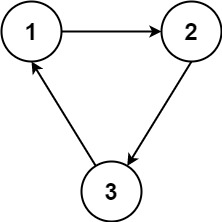Input: n = 3, relations = [[1,2],[2,3],[3,1]] Output: -1 Explanation: No course can be studied because they are prerequisites of each other.

Constraints:

``````1 <= n <= 5000
1 <= relations.length <= 5000
relations[i].length == 2
1 <= prevCoursei, nextCoursei <= n
prevCoursei != nextCoursei
All the pairs [prevCoursei, nextCoursei] are unique.
``````

• code
``````class Solution:
def minimumSemesters(self, n: int, relations: List[List[int]]) -> int:
indegree = {i:0 for i in range(1, n+1)}
graph = defaultdict(list)
for pre, nex in relations:
indegree[nex] += 1
graph[pre].append(nex)

q = deque([i for i in indegree if indegree[i] == 0])
step = 0
left = n
while q:
step += 1
for _ in range(len(q)):
cur = q.popleft()
left -= 1
for nex in graph[cur]:
indegree[nex] -= 1
if indegree[nex] == 0:
q.append(nex)
return step if left == 0 else -1
``````

#### Minimum Height Trees - LeetCode

A tree is an undirected graph in which any two vertices are connected by exactly one path. In other words, any connected graph without simple cycles is a tree. Given a tree of n nodes labelled from 0 to n - 1, and an array of n - 1 edges where edges[i] = [ai, bi] indicates that there is an undirected edge between the two nodes ai and bi in the tree, you can choose any node of the tree as the root. When you select a node x as the root, the result tree has height h. Among all possible rooted trees, those with minimum height (i.e. min(h)) are called minimum height trees (MHTs). Return a list of all MHTs' root labels. You can return the answer in any order. The height of a rooted tree is the number of edges on the longest downward path between the root and a leaf.

Example 1:Input: n = 4, edges = [[1,0],[1,2],[1,3]] Output:  Explanation: As shown, the height of the tree is 1 when the root is the node with label 1 which is the only MHT. Example 2: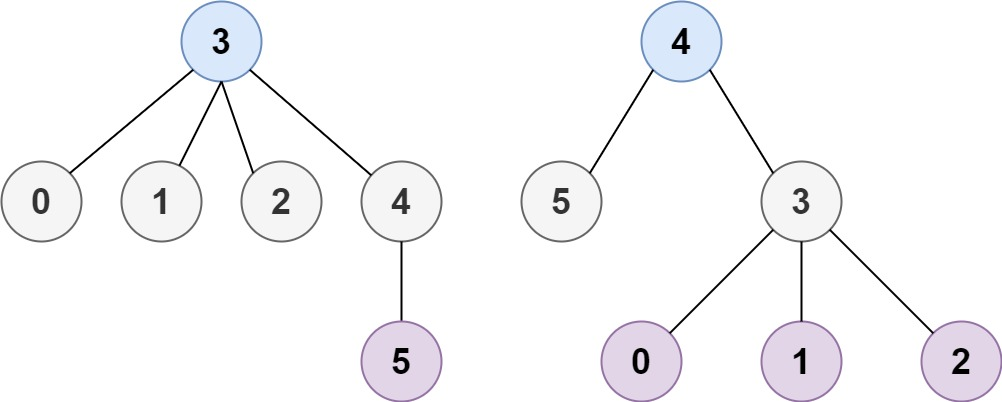Input: n = 6, edges = [[3,0],[3,1],[3,2],[3,4],[5,4]] Output: [3,4] Example 3: Input: n = 1, edges = [] Output:  Example 4: Input: n = 2, edges = [[0,1]] Output: [0,1]

Constraints:

1 <= n <= 2 * 104 edges.length == n - 1 0 <= ai, bi < n ai != bi All the pairs (ai, bi) are distinct. The given input is guaranteed to be a tree and there will be no repeated edges. C

• code
``````class Solution {
public List<Integer> findMinHeightTrees(int n, int[][] edges) {

// base cases
if (n <= 2) {
ArrayList<Integer> centroids = new ArrayList<>();
for (int i = 0; i < n; i++)
return centroids;
}

// Build the graph with the adjacency list
List<List<Integer>> neighbors = new ArrayList<>();
for (int i = 0; i < n; i++)

for (int[] edge : edges) {
int start = edge, end = edge;
}

// Initialize the first layer of leaves
ArrayList<Integer> leaves = new ArrayList<>();
for (int i = 0; i < n; i++)
if (neighbors.get(i).size() == 1)

// Trim the leaves until reaching the centroids
int remainingNodes = n;
while (remainingNodes > 2) {
remainingNodes -= leaves.size();
ArrayList<Integer> newLeaves = new ArrayList<>();

// remove the current leaves along with the edges
for (Integer leaf : leaves) {
// the only neighbor left for the leaf node
// Integer neighbor = neighbors.get(leaf).iterator().next();
Integer neighbor = neighbors.get(leaf).get(0);
// remove the edge along with the leaf node
neighbors.get(neighbor).remove(leaf);
if (neighbors.get(neighbor).size() == 1)
}

// prepare for the next round
leaves = newLeaves;
}

// The remaining nodes are the centroids of the graph
return leaves;
}
}
``````
• code
``````class Solution:
def findMinHeightTrees(self, n: int, edges: List[List[int]]) -> List[int]:
if n <= 2: return list(range(n))

adja = [[] for i in range(n)]

for i, j in edges:

leaves = [] # outmost
for node, nei in enumerate(adja):
if len(nei) == 1:
leaves.append(node)

nodeleft = n
while nodeleft > 2:
# remove leaves
nodeleft -= len(leaves)

newleaves = []
while leaves:
cur = leaves.pop()
only_nei = adja[cur].pop() # cur leaf's only nei